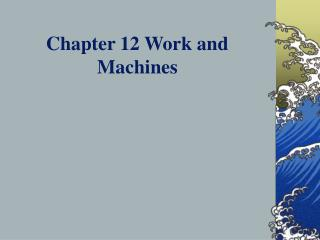# Chapter 12 Work and Machines - PowerPoint PPT PresentationDownload PresentationChapter 12 Work and Machines

Chapter 12 Work and MachinesDownload Presentation## Chapter 12 Work and Machines

- - - - - - - - - - - - - - - - - - - - - - - - - - - E N D - - - - - - - - - - - - - - - - - - - - - - - - - - -
##### Presentation Transcript

1. Chapter 12 Work and Machines

2. Section 1 - What is work? • Work - The product of force and distance when a force is used to move an object. • No work without motion • Work = Force x Distance • work units - Joules (J) • Problems pg 376 Chapter 12

3. Section 2 - Mechanical Advantage and Efficiency • Machine - • A device that changes the amount of force exerted or the direction in which force is exerted. • A device with which you can do work in a way that is easier or more effective. • A machine makes work easier by changing the amount of force you exert, the distance over which you exert your force, or the direction in which you exert your force. Chapter 12

4. Overhead - input/output force pg.379 • Increase force, decrease distance • Increase distance, decrease force • Mechanical advantage (MA) = output force/input force • Friction reduces mechanical advantage • Efficiency = output work/input work x 100% Chapter 12

5. Section 3 - Simple Machines • Six types of machines • Inclined plane • Wedge • Screw • Lever • Wheel & axle • Pulley Chapter 12

6. Inclined Plane • Less force exerted over longer distance Chapter 12

7. Wedge • Less force exerted over longer distance Chapter 12

8. Screw • Inclined plane wrapped around a cylinder • Less force Chapter 12

9. Lever • Fulcum - the fixed point that a lever pivots around Chapter 12

10. 3 Types of Levers Chapter 12

11. Wheel and Axle • Less force exerted over longer distance • Doorknob Chapter 12

12. Pulley • Change amount and direction of input force Chapter 12

13. Section 4 - Machines in the human body • Lifting levers - most of the machines in your body are levers that consist of bones and muscles. • Overhead -pg.401 Levers in the body • Wedges - front teeth (incisors) • Break toothpick in fingers Chapter 12

14. work, machine, efficiency, mechanical advantage, inclined plane, wedge, screw, lever, fulcrum, wheel and axle, pulley Ch. 12 Vocabulary Chapter 12